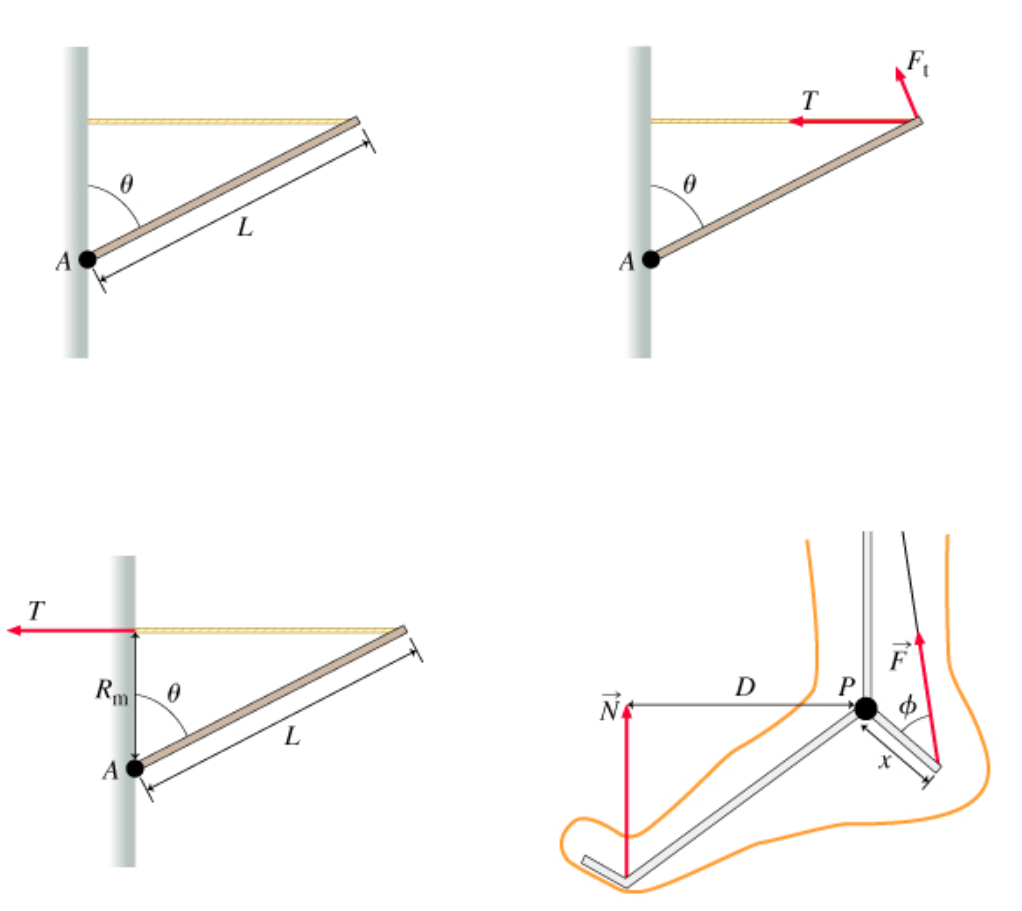# Problem: Learning Goal: To understand the two most common procedures for finding torques when the forces and displacements are all in one plane: the moment arm method and the tangential force method. The purpose of this problem is to give you further practice finding torques in two-dimensional situations. In this case it is overkill to use the full cross product definition of the torque because the only nonzero component of the torque is the component perpendicular to the plane containing the problem. There are two common methods for finding torque in a two-dimensional problem: the tangential force method and the moment arm method. Both of these methods will be illustrated in this problem. Throughout the problem, torques that would cause counterclockwise rotation are considered to be positive. Consider a uniform pole of length L, attached at its base (via a pivot) to a wall. The other end of the pole is attached to a cable, so that the pole makes an angle θ with respect to the wall, and the cable is horizontal. The tension in the cable is T. The pole is attached to the wall at point A. (Figure 1)a. Find the magnitude of the torque τ on the pole, about point A, due to the tension in the rope. Express your answer in terms of T, L, and θ.b. Find τN, the torque about point P due to the normal force. Express your answer in terms of N and any of the other quantities given in the figure.c. Find τF, the torque about point P due to the force applied by the Achilles' tendon. Express your answer in terms of F, ϕ, and x.

###### FREE Expert Solution

a.

Torque is given as:

τ = Force × length of the lever arm.

Force here is the tension, T.

The length of the lever arm is Lcosθ

80% (476 ratings)###### Problem Details

Learning Goal: To understand the two most common procedures for finding torques when the forces and displacements are all in one plane: the moment arm method and the tangential force method. The purpose of this problem is to give you further practice finding torques in two-dimensional situations. In this case it is overkill to use the full cross product definition of the torque because the only nonzero component of the torque is the component perpendicular to the plane containing the problem. There are two common methods for finding torque in a two-dimensional problem: the tangential force method and the moment arm method. Both of these methods will be illustrated in this problem. Throughout the problem, torques that would cause counterclockwise rotation are considered to be positive. Consider a uniform pole of length L, attached at its base (via a pivot) to a wall. The other end of the pole is attached to a cable, so that the pole makes an angle θ with respect to the wall, and the cable is horizontal. The tension in the cable is T. The pole is attached to the wall at point A. (Figure 1)

a. Find the magnitude of the torque τ on the pole, about point A, due to the tension in the rope. Express your answer in terms of T, L, and θ.

b. Find τN, the torque about point P due to the normal force. Express your answer in terms of N and any of the other quantities given in the figure.

c. Find τF, the torque about point P due to the force applied by the Achilles' tendon. Express your answer in terms of F, ϕ, and x.